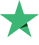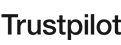Şu anda izleniyor
82 Öğrenciler
121 Ders
Başlangıç seviyesi

#### Ne Öğreneceksiniz?

• You'll know how and why use R for data science and machine learning You'll get practical tips and advise on Data Scientist's career You'll work on practical tasks and examine case studies to fully understand the fundamentals of R programming You'll be able to use the core features and packages of R

#### Müfredat

22sa 31dk
02:40
##### Bölüm 2: Introduction to Data Science
56:59
Introduction To Data Scientist
12:31
How to switch your career into ML part 1
14:56
How to switch your career into ML part 2
03:31
##### Bölüm 3: Course Curriculum Overview
22:41
Course Curriculum Overview
22:41
##### Bölüm 4: Introduction to R
26:15
Introduction to R
15:07
Setting up R
11:08
##### Bölüm 5: R Programming
55:14
R Operator
13:45
R Conditional Statement & Loop
12:02
R Programming - R Function #1
13:20
R Programming - R Function #2
10:10
R Programming - R Function #3
05:57
##### Bölüm 6: R Data Structure
49:23
An Introduction of R Data Structure + Vector
11:13
Matrix, Array and Data Frame
14:37
A Deep Drive to R Data Frame
13:02
Factor
04:12
R Data Structure - List
06:19
##### Bölüm 7: Import and Export in R
32:16
Import CSV Data in R
09:26
Import Text Data in R
03:19
Import Excel, Web Data in R
12:47
Export Data in R - Text
02:37
Export Data in R - CSV & Excel
04:07
##### Bölüm 8: Data Manipulation
1:33:49
Data Manipulation - Apply Function
13:15
Data Manipulation - select
11:46
Data Manipulation - mutate
14:28
Data Manipulation - filter
14:11
Data Manipulation - arrange
09:38
Data Manipulation - Pipe Operator
08:30
Data Manipulation - group by
11:26
Data Manipulation - Date
10:35
##### Bölüm 9: Data Visualization
2:10:04
Introduction to Data Visualiztion & Scatter Plot
12:01
Data Visualization - mfrow
07:37
Data Visualization - pch
12:30
Data Visualization - Color
01:19
Data Visualization - Line Chart
03:21
Data Visualization - Bar Plot
07:05
Data Visualization - Pie Chart
06:43
Data Visualization - Histogram
07:06
Data Visualization - Density Plot
02:26
Data Visualization - Box Plot
05:01
Data Visualization - Mosaic Plot and Heat Map
07:59
Data Visualization - 3D Plot
10:39
Correlation Plot and Word Cloud
09:02
Data Visualization - ggplot2 Part 1
14:03
Data Visualization - ggplot2 Part 2
08:08
Data Visualization - ggplot2 Part 3
15:04
##### Bölüm 10: Introduction To Statistics
2:02:57
Introduction To Statistics Part 1
13:25
Introduction To Statistics Part 2
08:53
Introduction To Statistics Part 3
14:55
Introduction To Statistics Part 4
04:15
Introduction To Statistics Part 5
15:10
Introduction To Statistics Part 6
08:21
Introduction To Statistics Part 7
15:04
Introduction To Statistics Part 8
10:45
Introduction To Statistics Part 9
10:24
Introduction To Statistics Part 10
14:34
Introduction To Statistics Part 11
07:11
##### Bölüm 11: Hypothesis Testing
41:58
Hypothesis Testing Part 1
10:08
Hypothesis Testing Part 2
11:28
Hypothesis Testing Part 3
14:21
Hypothesis Testing Part 4
06:01
##### Bölüm 12: Hypothesis Testing in Practice
2:14:01
Hypothesis Testing in Practice Part 1
15:04
Hypothesis Testing in Practice Part 2
09:36
Hypothesis Testing in Practice Part 3
14:16
Hypothesis Testing in Practice Part 4
12:36
Hypothesis Testing in Practice Part 5
10:29
Hypothesis Testing in Practice Part 6
13:46
Chi Square Part 1
11:19
Chi Square Part 2
14:57
ANOVA Part 1
12:30
ANOVA Part 2
14:20
What we discussed in the chapter so far?
05:08
##### Bölüm 13: Machine Learning Toolbox
26:31
Machine Learning Toolbox Part 1
14:00
Machine Learning Toolbox Part 2
12:31
12:51
12:51
##### Bölüm 15: Data Pre-Processing
1:24:14
Data Pre-Processing part 1
14:45
Data Pre-Processing part 2
14:29
Data Pre-Processing part 3
10:25
Data Pre-Processing part 4
09:39
Data Pre-Processing part 5
12:33
Data Pre-Processing part 6
07:19
Data Pre-Processing part 7
15:04
##### Bölüm 16: Supervised Learning : Regression
3:06:14
Linear Regression part 1
11:47
Linear Regression part 2
14:23
Linear Regression part 3
20:21
Linear Regression part 4
19:02
Linear Regression part 5
25:00
Linear Regression part 6
15:02
Linear Regression part 7 - Correlation Part 1
14:31
Linear Regression part 7 - Correlation Part 2
13:44
Linear Regression part 8 - Stepwise Regression
12:52
Linear Regression part 9 - Stepwise Regression
16:03
Linear Regression part 10 - Dummy Variable
12:34
Linear Regression part 11 - Non Linear
10:55
##### Bölüm 17: Classification Overview
13:29
Classification Overview
13:29
##### Bölüm 18: Logistic Regression
1:11:26
Logistics Regression Intuition
14:04
R Code Implementation Part 1
05:09
R Code Implementation Part 2
10:37
Model Evaluation
12:28
Telecom Churn Case Study
22:27
Summary
06:41
##### Bölüm 19: K-NN Study
40:13
K-NN Intuition
13:26
K-NN R Code Implementation
12:48
K-NN Case Study
13:59
##### Bölüm 20: SVM Study
45:18
SVM - Intuition
08:44
SVM - R Code Implementation
08:22
SVM - Model Tuning
09:00
SVM - Telecom Case Study
07:56
SVM - Non Separable Case and Pros and Cons
07:27
SVM Chapter summary
03:49
##### Bölüm 21: Naive Bayes
36:46
Naive Bayes - Intuition
19:56
Naive Bayes - R Code Implementation
08:25
Naive Bayes - Case Study
08:25
##### Bölüm 22: Decision Tree
1:06:18
Decision Tree Intuition
14:54
Decision Tree - How it works
07:40
Decision Tree - R Code Implementation
13:44
Decision Tree - Pruning
15:36
Decision Tree - Case Study
14:24

#### Gereksinimler

• No prior knowledge is required to enrol this course Software and data needed in the course will be provided for free

Yorumlar 15
Öğrenciler 1,597
Geniş kitle
Dersler 0

#### BitDegree için yapılan yorumlar

Öğrencilerimiz Mükemmel diyor
9.5 / 10